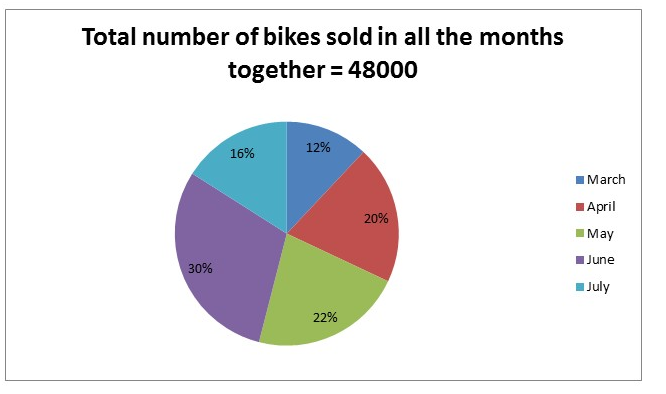# RRB PO Prelims Quantitative Aptitude Questions 2021 (Day-08)

Dear Aspirants, Our IBPS Guide team is providing new series of Quantitative Aptitude Questions for RRB PO Prelims 2021 so the aspirants can practice it on a daily basis. These questions are framed by our skilled experts after understanding your needs thoroughly. Aspirants can practice these new series questions daily to familiarize with the exact exam pattern and make your preparation effective.

Start Quiz

Pie chart

Directions (01-05): Study the following information carefully and answer the questions given below.

The given pie chart shows the number of bike sold in five different months.1) If the ratio of the number of bike sold in April to August is 8:7, how many bikes sold in August?

A.8200

B.8400

C.8600

D.8500

E.8800

2) If total number of bikes manufactured in March is 8800, then find the ratio of the number of sold to unsold bikes in March?

A.40:21

B.32:17

C.33:19

D.35:18

E.36:19

3) What is the average number of bike sold in March, June and July?

A.9280

B.9450

C.9370

D.9560

E.9670

4) If the ratio of the number of sold to unsold bikes in May is 11:9, then find the number of unsold bikes in May?

A.8460

B.8570

C.8750

D.8640

E.8340

5) The number of bikes sold in July is what percent of the number of bikes sold in April?

A.70%

B.80%

C.75%

D.85%

E.90%

Time, speed and distance

6) Ratio of the speed of car to bike is 3:2 and the car covers 480 km in 8 hours. If the time taken by bike covers x km in 3 hours more than the time taken by the car covers the same distance, then find the value of x?

A.320 km

B.300 km

C.360 km

D.400 km

E.None of these

Probability

7) A bag contains 3 yellow, 5 pink and 4 white balls. If two balls are drawn at random, then what is the probability that balls are either yellow or pink?

A.13/66

B.2/11

C.1/5

D.7/33

E.None of these

Boat and stream

8) The speed of the boat in still water is 200% more than the speed of the stream. If the boat covers d km along with stream in 6 hours and the same boat covers the same distance against stream in 12 hours, find the downstream speed of the boat?

A.20 kmph

B.24 kmph

C.32 kmph

D.16 kmph

E.Cannot be determined

Profit and loss

9) The cost price of four mobiles is equal to the cost price of 3 laptops. If the cost price of 5 laptops and 7 mobiles is Rs.12300, then what is the cost of 12 mobiles and 7 laptops together?

A.Rs.19200

B.Rs.18800

C.Rs.17900

D.Rs.21000

E.None of these

Trains

10) Train A crosses a stationary train in 36 seconds and crosses a pole in 14.4 seconds. If the length of train A is 240 m, then find the length of stationary train?

A.300 m

B.160 m

C.440 m

D.360 m

E.460 m

Number of bike sold in April = 20/100 * 48000 = 9600

Number of bike sold in August = 9600 * 7/8 = 8400

Number of sold bikes in March = 12/100 * 48000 = 5760

Number of unsold bikes in March = 8800 – 5760 = 3040

Required ratio = 5760:3040

= 288:152

= 36:19

Required average = (12 + 30 + 16)/300 * 48000 = 9280

Number of sold bikes in May = 22/100 * 48000 = 10560

Number of unsold bikes in May = 10560 * 9/11 = 8640

Required percentage = 16/20 * 100 = 80%

Speed of car = 480/8 = 60 kmph

Speed of the bike = 2/3 * 60 = 40 kmph

x/40 – x/60 = 3

3x – 2x = 3 * 120

x = 360 km

Required probability = (3C2 + 5C2)/12C2

= 13/66

Distance = d

Speed of stream = x

Speed of boat = x * 300/100 = 3x

d/4x = 6

d/(3x – x) = 12

CP of a mobile = x

CP of a laptop = y

4x = 3y

CP of 5 laptops = 5 * (4x/3) = 20x/3

20x/3 + 7x = 12300

41x = 12300 * 3

x = 900

CP of a laptop = 4 * 900/3 = 1200

Required sum = 12 * 900 + 7 * 1200

= Rs.19200

Speed of train A = x

240 = x * 5/18 * 14.4

x = 60 kmph

240 + Length of stationary train = 60 * (5/18) * 36

Length of stationery train = 360 m